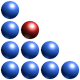# How Structure Leads to Information

The importance of structure in physics and other branches of science cannot be overstated. Stucture is knowledge, structure is understanding. By structure we intend a map, or a graph, which reflects how information travels within a given system. In other words, we looking at the topology of all the interdependencies between the various actors, agents, or simply data channels, which compose or describe a system. Without structure, information cannot flow.

An example of structure is shown below. It has the form of a map. The squares represent agents (or data channels) while the black dots represent the said interdependencies. In general, the map’s structure changes in time as the underlying system evolves.

In the above map there are 29 variables which describe the system in question. If the veriables weren’t interdependent (i.e. there would be no map), the total amount of information contained in the 29 variables would simply be the sum of all the information carried by each variable and given by Shannon’s equation:

Now, complexity, defined as C=f(S; E), not only captures and quantifies the intensity of the dynamic interactions between Structure and Entropy, it also measures the amount of information that is the result of structure. In fact, entropy – the ‘E’ in the complexity equation – is already a measure of information. However, the ‘S’ holds additional information which is ‘contained’ in the structure of the interdependency map. In other words, S encodes additional information to that provided by the Shannon equation. In the example in 29 dimensions shown above the information breakdown is as follows:

• Shannon’s information (in all 29 channels) = 60.25 bits
• Information due to structure = 284.80 bits
• Total information = 345.05 bits

In this example, structure furnishes nearly five times more information than the sum of the information content of each dimension. For higher dimensions the ratio can be significantly higher.

Complexity, therefore, is not just a measure of how intricate, or sophisticated, something is. It has a deeper significance in that it defines the topology of information flow and, therefore, of the processes which make Nature work. In addition, it quantifies the total amount of information within a system, in particular that emanating from the presence of structure.

www.ontonix.com1.